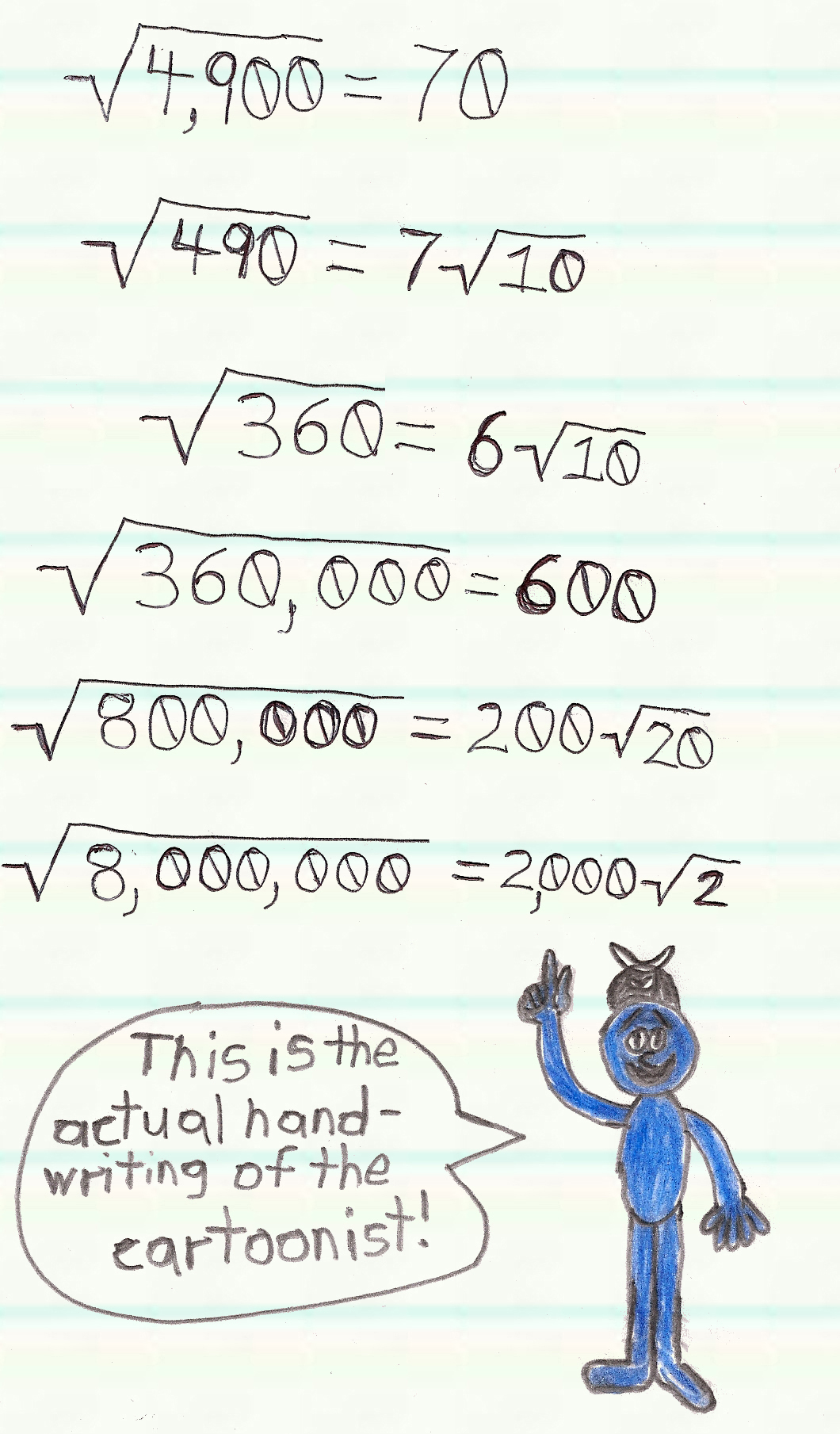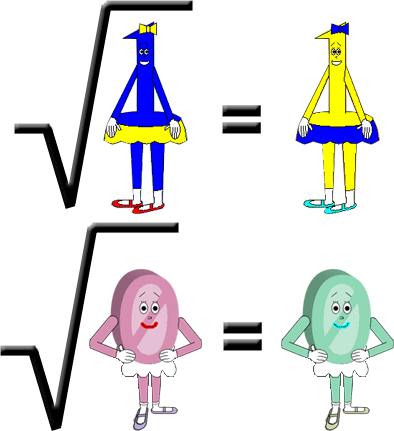# Square Root Squabble 2

## There's something quite interesting about the square roots of integers with several zeroes in them! If you observe the image below:If the integer has an even number of zeroes, then the square root will have half as many zeroes! If the integer has an odd number of zeroes, then...you can write the square root as an integer multiplied by the square root of 10; you should already know that most integers have irrational square roots. However, according to the lower 2 examples in the image, sometimes you might have to multiply by the square root of an integer other than 10. (It has something to do with factors) By the way, make sure you pick a multiple of 10 to put under the radical sign to the left of the equal sign or this math trick will fail to work! Also, if you multiply 10 by a prime number, this math trick won't work! (Except with the only even prime number 2 since the square root of 20 = 2 × the square root of 5. It also works with 5; the square root of 50 = 5 × the square root of 2.)